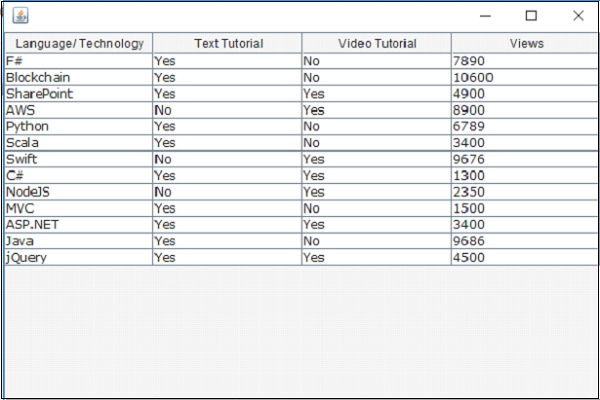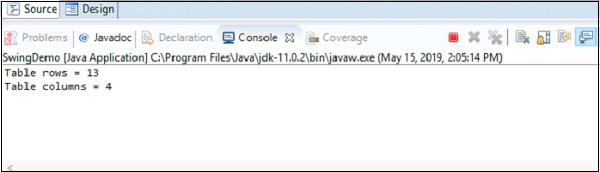# How to get the number of rows and columns of a JTable in Java Swing

Java 8Object Oriented ProgrammingProgrammingSwing

To count the number of rows of a table, use the getRowCount() method −

table.getRowCount()

To count the number of columns of a table, use the getColumnCount() method −

table.getColumnCount()

The following is an example to get the number of rows and columns of a JTable −

## Example

package my;
import java.awt.Font;
import javax.swing.JFrame;
import javax.swing.JScrollPane;
import javax.swing.JTable;
import javax.swing.table.DefaultTableModel;
public class SwingDemo {
public static void main(String[] argv) throws Exception {
DefaultTableModel tableModel = new DefaultTableModel();
JTable table = new JTable(tableModel);
// prevent from resizing
tableModel.addRow(new Object[] { "F#", "Yes", "No", "7890"});
tableModel.addRow(new Object[] { "Blockchain", "Yes", "No", "10600"});
tableModel.addRow(new Object[] { "SharePoint", "Yes", "Yes", "4900"});
tableModel.addRow(new Object[] { "AWS", "No", "Yes", "8900"});
tableModel.addRow(new Object[] { "Python", "Yes", "No", "6789"});
tableModel.addRow(new Object[] { "Scala", "Yes", "No", "3400"});
tableModel.addRow(new Object[] { "Swift", "No", "Yes", "9676"});
tableModel.addRow(new Object[] { "C#", "Yes", "Yes", "1300"});
tableModel.addRow(new Object[] { "NodeJS", "No", "Yes", "2350"});
tableModel.addRow(new Object[] { "MVC", "Yes", "No", "1500"});
tableModel.addRow(new Object[] { "ASP.NET", "Yes", "Yes", "3400"});
tableModel.addRow(new Object[] { "Java", "Yes", "No", "9686"});
tableModel.addRow(new Object[] { "jQuery", "Yes", "Yes", "4500"});
System.out.println("Table rows = "+table.getRowCount());
System.out.println("Table columns = "+table.getColumnCount());
Font font = new Font("Verdana", Font.PLAIN, 12);
table.setFont(font);
JFrame frame = new JFrame();
frame.setSize(600, 400);
frame.setVisible(true);
}
}

This will produce the following output displaying the table with rows and columns −

## OutputThe count of rows and columns are displayed in the console as shown below −